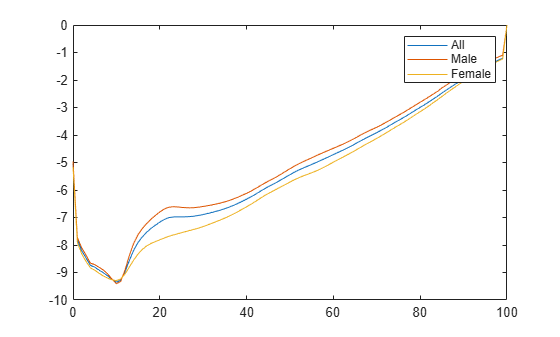# lifetablefit

Calibrate life table from survival data with parametric models

## Syntax

``````[a,elx] = lifetablefit(x,lx)``````
``````[a,elx] = lifetablefit(___,lifemodel,objtype,interpmethod,a0)``````

## Description

example

``````[a,elx] = lifetablefit(x,lx)``` calibrates a life table, `x`, from survival data, `lx`, using parametric models.```

example

``````[a,elx] = lifetablefit(___,lifemodel,objtype,interpmethod,a0)``` calibrates a life table, `x`, from survival data, `lx`, using parametric models using optional arguments for `lifemodel`, `objtype`, `interpmethod`, and `a0`.```

## Examples

collapse all

Load the life table data file.

`load us_lifetable_2009`

Calibrate the life table from survival data using the default `heligman-pollard` parametric model.

```[a,elx] = lifetablefit(x,lx); display(a)```
```a = 8×3 0.0005 0.0006 0.0004 0.0592 0.0819 0.0192 0.1452 0.1626 0.1048 0.0007 0.0011 0.0007 6.2849 6.7639 1.1037 24.1387 24.2894 53.1811 0.0000 0.0000 0.0000 1.0971 1.0987 1.1100 ```
`display(elx(1:20,:))`
``` 1.0e+05 * 1.0000 1.0000 1.0000 0.9937 0.9931 0.9943 0.9932 0.9926 0.9939 0.9930 0.9923 0.9936 0.9928 0.9921 0.9935 0.9926 0.9920 0.9933 0.9925 0.9919 0.9932 0.9924 0.9918 0.9931 0.9923 0.9917 0.9930 0.9922 0.9916 0.9929 0.9921 0.9914 0.9928 0.9920 0.9913 0.9927 0.9919 0.9912 0.9926 0.9917 0.9910 0.9924 0.9915 0.9908 0.9923 0.9913 0.9905 0.9921 0.9910 0.9901 0.9919 0.9906 0.9896 0.9917 0.9901 0.9890 0.9914 0.9895 0.9882 0.9912 ```

Plot the `qx` series and display the legend. The series `qx` is the conditional probability that a person at age x will die between age x and the next age in the series

```plot(x,log(qx)) legend(series)```## Input Arguments

collapse all

Increasing ages for raw data, specified as a `N` vector for nonnegative integers.

Data Types: `double`

Collection of `num` discrete survival counts, specified as an `N`-by-`num` matrix. The input `lx` series is the number of people alive at age x, given 100,000 alive at birth. Values of `0` or `NaN` in the input table `lx` are ignored.

Data Types: `double`

(Optional) Parametric mortality model type, specified as a character vector with one of the following values:

• `'heligman-pollard'` — Eight-parameter Heligman-Pollard model (version 1), specified in terms of the discrete hazard function:

`$\frac{q\left(x\right)}{1-q\left(x\right)}={A}^{{\left(x+B\right)}^{C}}+D\mathrm{exp}\left(-E{\left(\mathrm{log}\frac{x}{F}\right)}^{2}\right)+G{H}^{X}$`

for ages x`0`, with parameters A, B, C, D, E, F, G, H`0`.

• `'heligman-pollard-2'` — Eight-parameter Heligman-Pollard model (version 2), specified in terms of the discrete hazard function:

`$\frac{q\left(x\right)}{1-q\left(x\right)}={A}^{{\left(x+B\right)}^{C}}+D\mathrm{exp}\left(-E{\left(\mathrm{log}\frac{x}{F}\right)}^{2}\right)+\frac{G{H}^{X}}{1+G{H}^{X}}$`

for ages x`0`, with parameters A, B, C, D, E, F, G, H`0`.

• `'heligman-pollard-3'` — Eight-parameter Heligman-Pollard model (version 3), specified in terms of the discrete hazard function:

`$q\left(x\right)={A}^{{\left(x+B\right)}^{C}}+D\mathrm{exp}\left(-E{\left(\mathrm{log}\frac{x}{F}\right)}^{2}\right)+G{H}^{X}$`

for ages x`0`, with parameters A, B, C, D, E, F, G, H`0`.

• `'gompertz'` — Two-parameter Gompertz model, specified in terms of the continuous hazard function:

`h(x) = A exp(Bx)`
for ages x`0`, with parameters A, B`0`.

• `'makeham'` — Three-parameter Gompertz-Makeham model, specified in terms of the continuous hazard function:

`h(x) = A exp(Bx) + C`
for ages x`0`, with parametersA, B, C`0`.

• `'siler'` — Five-parameter Siler model, specified in terms of the continuous hazard function:

`h(x) = A exp(Bx) + C + D exp(-Ex)`
for ages x`0`, with parameters A, B, C, D, E`0`.

Data Types: `char`

(Optional) Objective for nonlinear least-squares estimation, specified as a character vector with the following values:

• `'ratio'` — Given raw data qx and model estimates ${\stackrel{^}{q}}_{x}$ for x = `1`, ... , N, the first objective (which is the preferred objective) has the form

`$\Phi ={\sum _{x=1}^{N}\left(1-\frac{{\stackrel{^}{q}}_{x}}{{q}_{x}}\right)}^{2}$`

• `'logratio'` — Given raw data qx and model estimates ${\stackrel{^}{q}}_{x}$ for x = `1`, ... , N, the second objective has the form

`$\Phi ={\sum _{x=1}^{N}\left(\mathrm{log}\left({\stackrel{^}{q}}_{x}\right)-\mathrm{log}\left({q}_{x}\right)\right)}^{2}$`

Data Types: `char`

(Optional) Interpolation method to use for abridged life table inputs, specified as a character vector with the following values:

• `'cubic'` — Cubic interpolation that uses `'pchip'` method in `interp1`.

• `'linear'` — Linear interpolation.

• `'none'` — No interpolation.

Note

If the ages in `x` are not consecutive years and interpolation is set to `'none'`, then the estimates for the parameters are suitable only for the age vector `x`.

If you use the parameter estimates to compute life table values for arbitrary years, interpolate using the default `'cubic'` method.

Interpolation with abridged life tables forms internal interpolated full life tables, which usually improves model fits.

Data Types: `char`

(Optional) Initial parameter estimate to be applied to all series, specified as a `numparam` vector. This vector must conform to the number of parameters in the model specified using the `lifemodel` argument.

Data Types: `double`

## Output Arguments

collapse all

Parameter estimates for each `num` series, returned as a `numparam`-by-`num` matrix.

Estimated collection of `num` standardized survivor series, returned as an `N`-by-`num` matrix. The `elx` output series is the number of people alive at age x, given 100,000 alive at birth. Values of `0` or `NaN` in the input table `lx` are ignored.

 Arias, E. “United States Life Tables.” National Vital Statistics Reports, U.S. Department of Health and Human Services. Vol. 62, No. 7, 2009.

 Carriere, F. “Parametric Models for Life Tables.” Transactions of the Society of Actuaries. Vol. 44, 1992, pp. 77–99.

 Gompertz, B. “On the Nature of the Function Expressive of the Law of Human Mortality, and on a New Mode of Determining the Value of Life Contingencies.” Philosophical Transactions of the Royal Society. Vol. 115, 1825, pp. 513–582.

 Heligman, L. M. A., and J. H. Pollard. “The Age Pattern of Mortality.” Journal of the Institute of Actuaries Vol. 107, Pt. 1, 1980, pp. 49–80.

 Makeham, W. M. “On the Law of Mortality and the Construction of Annuity Tables.” Journal of the Institute of Actuaries Vol. 8, 1860, pp. 301–310.

 Siler, W. “A Competing-Risk Model for Animal Mortality.” Ecology Vol. 60, pp. 750–757, 1979.

 Siler, W. “Parameters of Mortality in Human Populations with Widely Varying Life Spans.” Statistics in Medicine Vol. 2, 1983, pp. 373–380.